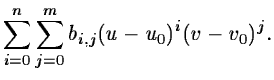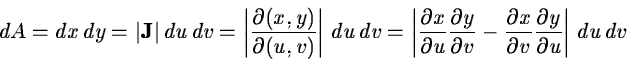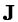Next: Image Manipulations Up: Raw to Calibrated Data Previous: Response Calibration

Geometric Corrections

Most imaging systems contain intrinsic geometric distortions. Although they can often be disregarded for small field corrections they must be applied when image tubes or dispersive elements (e.g. in spectrographs) are used. The actual form of the distortions is determined by observing a known grid of points or spectral lines. Normally, a power series is fitted to the point giving the coordinate transformation

 x = X(u,v) =(2.13) y = Y(u,v) =(2.14)

where (u0,u0) is an arbitrary reference point. The area of a pixel is changed by this transformation with an amount(2.15)

whereis the Jacobian determinant. The intensity values in the transformed frame must be corrected by this function so that the flux is maintained both locally and globally. A wavelength transformation for an image tube spectrum is shown in Figure 2.5 where both resulting spectra with and without flux correction are given.Although this is mathematically very simple, it gives significant numeric problems due to the finite size of pixels. The main problem is that one has to assume a certain distribution of flux inside a pixel e.g. constant. This assumption may affect the detailed local flux conservation and introduce high frequence error in the result. A further problem is the potential change of the noise distribution due to the interpolation scheme used. This can be solved be careful assignment of weight factors or by simply reducing the high frequence noise in the original frame by smoothing.Next: Image Manipulations Up: Raw to Calibrated Data Previous: Response Calibration
Petra Nass
1999-06-15